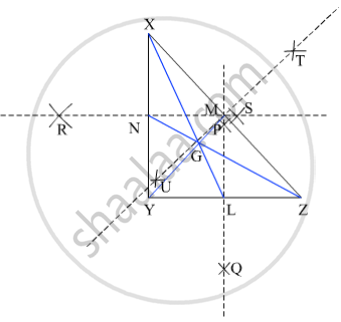SSC (English Medium) Class 8Maharashtra State Board
Share

# Draw a Right Angled δ Xyz. Draw Its Medians and Show Their Point of Concurrence by G. - SSC (English Medium) Class 8 - Mathematics

ConceptAltitudes of a Triangle

#### Question

Draw a right angled Δ XYZ. Draw its medians and show their point of concurrence by G.

#### SolutionSteps of construction :
(i) Draw a right angled ∆XYZ.
(ii) Draw the perpendicular bisector PQ of side YZ that intersect YZ at L.
(iii) Join XL. XL is the median to the side YZ.
(iv) Draw the perpendicular bisector TU of side ZX that intersect YZ at M.
(v) Join YM. YM is the median to side ZX.
(vi) Draw the perpendicular bisector RS of side XY that intersect XY at N.
(vii) Join ZN. ZN is the median to the side XY.
Hence, ∆XYZ is the required triangle in which medinas XL, YM and ZN to the sides YZ, ZX and XY respectively intersect at G.
The point G is the centroid of ∆XYZ.

Is there an error in this question or solution?

#### APPEARS IN

Balbharati Solution for Balbharati Class 8 Mathematics (2019 to Current)
Chapter 4: Altitudes and Medians of a triangle
Practice Set 4.1 | Q: 5 | Page no. 22
Solution Draw a Right Angled δ Xyz. Draw Its Medians and Show Their Point of Concurrence by G. Concept: Altitudes of a Triangle.
S